# NCERT Solutions for Chapter 4 Parts And Wholes Class 5 MathsParts And Wholes Questions and Answers

 Chapter Name Parts And Wholes NCERT Solutions Class CBSE Class 5 Textbook Name Maths Related Readings NCERT Solutions for Class 5NCERT Solutions for Class 5 Maths Summary of Parts And Wholes

Page No 50:

Question 1: Draw a rectangle of length 8 cm and width 6 cm. Divide it into three equal parts and complete the flag.

The top one-third of our flag is saffron (or orange). What is the colour of the middle one-third of the flag? Where will you draw the Ashoka chakra?

How much of the flag will you colour green?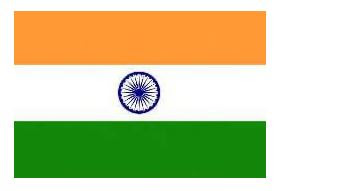The colour of middle one-third of the flag is white. The Ashoka chakra is drawn at the centre of the flag. One-third of the flag is coloured green.

Question 2: Now look at this flag. How much of it is black? ________

The green part of the flag can be written as ________  Is red less than one-third of the flag? Why?Total number of parts of the flag = 3 Now, we see that out of three equal parts, only one part of the flag is black. Thus, 13 of the flag is black.

We can also see that out of three parts of the flag, only one part is green. Thus, green part of the flag is 1/3 of the flag.

The red portion of the flag is less than 1/3 of the flag, because a white emblem is also present in the red portion of the flag.

Question 3: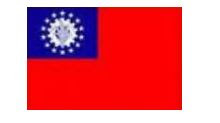This is the flag of Myanmar, our neighbour. Is blue more than one-fourth of the flag or less? Guess how much of the flag is red. Is it more than 1/2 ? Is it more than three-fourths?

The blue colour is present in less than one-fourth of the flag.

I think, red colour is present in more than three-fourth of the flag.

Page No 52:

Question 1: Draw a circle of radius 3 cm and cut it out. Divide the circle into 8 equal parts. Now each part is 18 of the circle. Colour 28 red, 18 oranges, 18 yellow etc. as shown here. Push a matchstick through the centre of the circle. Your magic top is ready. Spin it fast! What do you see? Can you see all the colours? Write what you see in your notebook.

Question 2:

Chocolate bar • Manju had a chocolate. She gave one-fourth of it to Raji, one-third to Sugatha and one-sixth to Sheela. She ate the remaining part. How many pieces of chocolate did each get?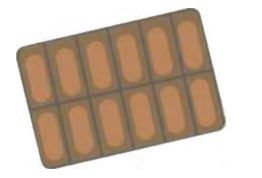• What part of the chocolate did Manju eat?

Total number of pieces in the chocolate bar = 12

Manju gives one-fourth of the chocolate to Raji. Number of pieces of chocolate given to Raji = 12 ÷

4 = 3

Thus, Raji got 3 pieces of chocolate. Now, Manju gives one-third of the chocolate to Sugatha. Number of pieces of chocolate given to Sugatha = 12÷ 3 =  4

Thus, Sugatha got 4 pieces of chocolate. Now, Manju gives one-sixth of the chocolate to Sheela. Number of pieces of chocolate given to Sheela = 12 ÷ 6=2 Thus, Sheela got 2 pieces of chocolate. Total number of pieces of chocolate given to Raji, Sugatha and Sheela 3+ 4+2 = 9 Total number of pieces of chocolate left in the bar = 12-9 = 3

As Manju ate the remaining part of the chocolate, she will get 3 pieces of chocolate. Part of the chocolate eaten by Manju = 14

Page No 53:

Question 1: Colour the hats Colour 1/3 of the hats red. Colour three-fifth hats blue. How many hats did you colour red? How many hats did you colour blue? What part of the hats are not coloured?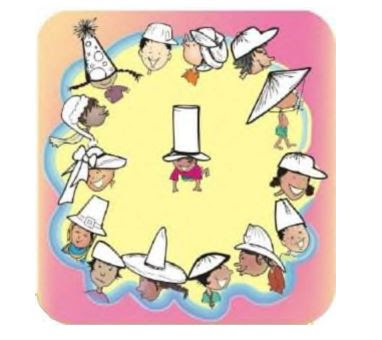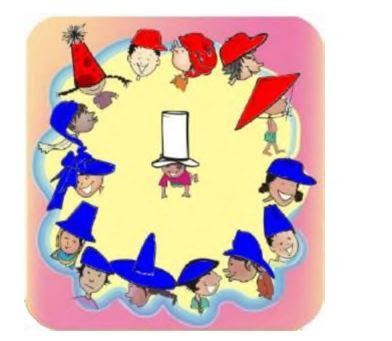Total number of hats = 15 It is given that we have to colour 13 of hats in red. Number of red coloured hats = 15 ÷ 3 =5

It is given that we have to colour 35 of the total hats in blue.

One-fifth of the total hats =15÷5=3 Number of blue coloured hats = 3

x3=9

Number of hats that are not coloured =1 Thus, part of hats that are not coloured = 1/15

Page No 55:

Question 1: Greedy Gatekeepers Remember Birbal, the clever minister of King Akbar? (Math-Magic Class IV. page 14) Do you know how he became a minister? Birbal was then a young boy living in a village. He was very clever and could write poetry. He thought he would try his luck in the King's court. So he took some of his poems and set off for the city. When he reached the outer gate of the palace, he was stopped by the gatekeeper. "Hey! Stop there! Where are you going?", shouted the gatekeeper. "I am a poet. I want to see King Akbar and show my poems to him", replied the poet. "Oh, you are a poet! The king is kind; he will surely give you a prize. I will let you in if you give me 110 of your prize". Young Birbal agreed since he had no other way. When he went in, the gatekeeper calculated "If he gets 100 gold coins I will get ________ coins". The poet came to a second gatekeeper. This gatekeeper also said, "I will let you in if you give me two-fifth of your prize". The poet agreed. The gatekeeper happily calculated, "The poet will get at least 100 gold coins so I will get ________ gold coins!" The poet reached the last gate. The gatekeeper said, "I will allow you to see the king only if you give me half of the prize that you get". The poet had no other way. He agreed and went inside. The gatekeeper thought, "Today is a great day. If he gets 100 gold coins I will get ________ gold coins. But if he gets 1000 coins â€ wow! I will get ________ ". The king was very happy with the poems and said, "Your work is very good. You can ask anything as your prize". "My Lord, I want 100 slaps". "What! 100 slaps? ________ ". The king was shocked â€. What happened after that? Complete the story. What part of the prize did the poet get?

The first gatekeeper thought that the king would give 100 gold coins to Birbal as a prize. The first gatekeeper demanded 110 of the prize that he would get from the king. So, number of gold coins that would be received by first gatekeeper = 100 ÷ 10 = 10

The second gatekeeper demanded 25 of the prize that Birbal would receive from the king. One-fifth of 100 = 100 ÷ 5 = 20

So, number of gold coins that would be received by second gatekeeper = 20 × 2=40

The third gatekeeper demanded half of the prize that Birbal would receive from the king. So, number of gold coins that would be received by third gatekeeper =100 ÷ 2=50

After listening to his poems, the king became happy. Then, Birbal requested the king to give him 100 slaps as a prize.

Hence, the third gatekeeper got 50 slaps, second gatekeeper got 40 slaps, and the first gatekeeper got 10 slaps. The poet got 0 slaps.

Page No 57:

Question 1: Make different patterns by colouring some squares in the grids B, C, D. What part of the grid did you colour? What part of the grid remained white? Write.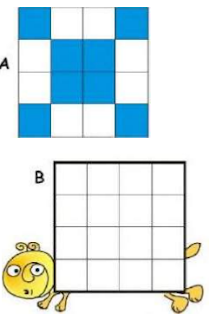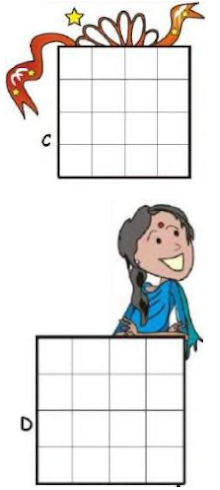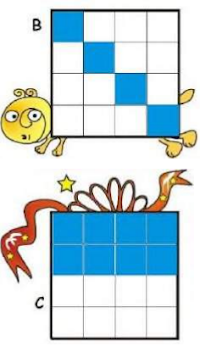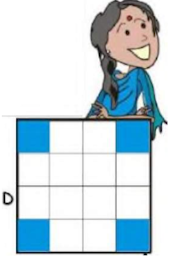Question 2: Look at grid A again. Is the grid coloured

(a) 1/2 blue, 1/2 white?

(b) 2/4 blue, 2/4 white?

(c) 3/8 blue, 5/8 white?

(d) 4/8 blue, 4/8 white?

Mark (×) on the wrong answer.

Total number of squares in grid A = 16

Number of squares that are blue-coloured = 8

Number of squares that are white-coloured = 8

Fraction of grid A that is blue-coloured = 1/2

Fraction of grid A that is white-coloured = 1/2

So,  1/2 of the grid A is blue and 1/2 of the grid A is white.

1/2 = 2/4 = 4/8

 a) 1/2 blue, 1/2 white b) 2/4 blue, 2/4 white c) 3/8 blue, 5/8 white (×) d) 4/8 blue, 4/8 white

Question 3: Draw grids of 16 squares and make patterns with

(a) 2/8 red, 1/2 yellow, 1/4 green

(b) 3/16 blue, 5/16 red, 1/2 yellow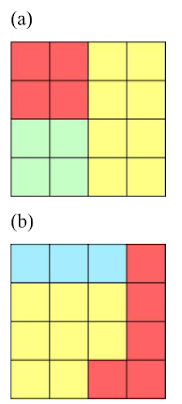Page No 58:

Question 1: Ramu's vegetable field has 9 equal parts. What vegetables does he grow?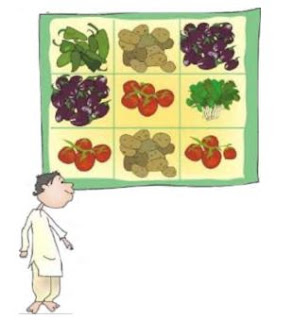(1) Which vegetable grows in the biggest part of his field? What part?

(2) On what part of the field does he grow potatoes?

(3) What part of the field is used to grow spinach? What part is used for brinjal?

(4) Now you write some questions by looking at this picture.

Ramu grows capsicum, brinjal, tomatoes, spinach and potatoes in his field.

1) Total number of parts of the vegetable field=9

Ramu grows tomatoes in the biggest part of his field. Part of field used for growing tomatoes = 3

Part of field used for growing tomatoes = 3/9 = 1/3

Ramu grows tomatoes in one-third of the field.

2) Part of field used for growing potatoes = 2

Total number of parts of the vegetable field = 9

Part of the field used for growing potatoes = 2/9

So, Ramu grows potatoes in two-ninth part of the field.

3) Total number of parts of the vegetable field = 9

Number of parts used for growing spinach = 1

So, part of the field used for growing spinach = 1/9

Number of parts of field used for growing brinjals = 2

So, part of the field used for growing brinjals = 2/9

Thus, Ramu grows spinach and brinjals in one-ninth and two-ninth part of the field respectively.

4)

(1) Which vegetables are grown in the least part of the field?

(2) What is the part of the field used to grow raddish and tomatoes together?

Disclaimer: The answer to part (4) of the question may vary from student to student. It is highly recommended that the students prepare the answer on their own. The answer provided here is for reference only.

Page No 59:

Question 1: Ramu wanted to give below vegetables to his friends. He gave Aboobacker one-fifth of these tomatoes and 1/3 of the potatoes. Srija got 2/5 of the tomatoes and 3/6 of the potatoes. Nancy got the rest of these vegetables. Circle Aboobacker's share in blue. Circle Srija's share in yellow.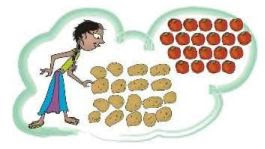·  How many potatoes and tomatoes did Nancy get?

Total number of tomatoes = 20 Total number of potatoes = 18

Aboobacker got one-fifth of the tomatoes, and one-third of the potatoes. Number of tomatoes with Aboobacker = 20 ÷ 5 = 4 Number of potatoes with Aboobacker = 18÷3=6

So, we will circle 4 tomatoes and 6 potatoes in blue to show Aboobacker's share. Srija got two-fifth of the tomatoes and three-sixth of the potatoes. Number of tomatoes with Srija = 2 x 4 = 8 One-sixth of 18 = 3 Number of potatoes with Srija = 3 × 3 = 9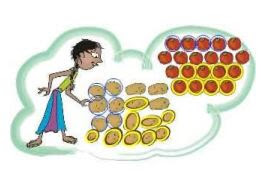Thus, Ramu gave 4 tomatoes to Aboobacker and 8 tomatoes to Srija. So, he gave a total of 12 tomatoes to Aboobacker and Srija.

Now, Ramu gave 6 potatoes to Aboobacker and 9 potatoes to Srija. So, he gave a total of 15 potatoes to Aboobacker and Srija.

Number of tomatoes left = 20-12=8

Number of potatoes left = 18-15=3

Thus, Nancy got 8 tomatoes and 3 potatoes.

Page No 60:

Question 1: The Card Puzzle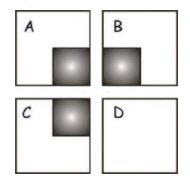(1) Divide the white area in square A into two equal parts. Got the answer? Was that easy? Now do the second question.

(2) Divide the white area in square B into three equal parts! That too is easy, isn't it? Now see the third question.

(3) Divide the white area in square C into four equal parts!! Is it a bit difficult? Don't worry, take your time. Only if you have given up, look for the answer. Here comes the last question.

(4) Divide the white area in square D into seven equal parts!!!! The world record for this is 7 seconds. But you can take minutes! Tired of thinking? Look for the answer on page 68. So was that difficult??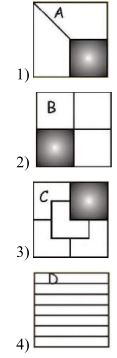Page No 61:

Question 1: What part of each shape is coloured? First guess the answer, then check.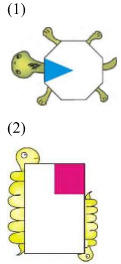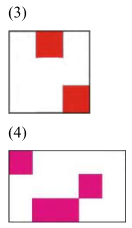Following are the guesses:

1) One-eighth of the shape is coloured.

2) One-sixth of the shape is coloured.

3) Two-ninth of the shape is coloured.

4) Four-fifteenth of the shape is coloured.

To cross-check our guess, we will divide each of the given shapes into equal parts of size of the coloured part as shown below: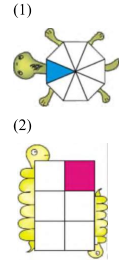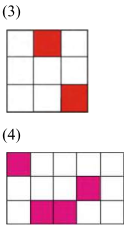On cross-checking, we now find that our guess is correct.

Page No 62:

Question 1: Look at the small triangle. What part of the square is it? How will you find this out?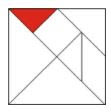We will divide the whole square into small triangles as shown in the adjoining figure.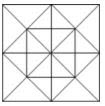We will get 16 such triangles.

Total number of triangles = 16

Number of coloured triangles = 1

Fraction of square coloured = 1/16

Thus, the coloured small triangle is one-sixteenth of the square.

Question 2: Coloured Parts Complete these

(1)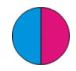This circle is divided into two equal parts. Out of _______ equal parts one part is coloured blue.

(2)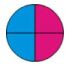Here the circle is divided into _______ equal parts. Out of _______ equal parts, _______

parts are coloured blue.

(3)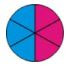Here the circle is __________________________________________

(4)Here the circle is __________________________________________

(1) The circle is divided into two equal parts. Out of two equal parts, one part is blue in colour.

(2) Here, the circle is divided into four equal parts. Out of four equal parts, two parts are blue-coloured.

(3) Here, the circle is divided into six equal parts. Out of six equal parts, three parts are blue-coloured.

(4) Here, the circle is divided into eight equal parts. Out of eight equal parts, four parts are blue-coloured.

So, we can say that,

1/2 = 2/4 = 3/6 = 4/8

Page No 63:

Question 1: Ramesh bought a piece of halwa for his children Ammu and Anu. He divided it equally for them.

• Each will get _________ part of halwa. "This piece is too big. We can't eat it", they said. So he divided the pieces into half again. Now how many pieces will Ammu get?
• What part of the halwa is it? _________"Make it even smaller, Dad" they asked. So he again cut the halwa into smaller pieces. "Ok, thank you, Dad." Now how many pieces will each get?
• What part of the halwa is each piece now?
• If Ramesh had cut the halwa into 6 equal parts how many pieces would each have got? Look at your answers for questions 1 to 4 and write = 1/2= ____= ____ =____ =____ =____

• When Ramesh divides the piece of halwa in two parts, then each child will get half part of halwa. Each will get 1/2 part of halwa.
• When each of the two pieces of halwa is divided into 2 equal parts, then there will be a total of 4 pieces of halwa. Now, each of the child gets 2 pieces of halwa.

Thus, Ammu will get 2 pieces of halwa.

Total number of pieces of halwa = 4

Number of pieces with Ammu = 2

Part of halwa that Ammu gets = 2/4 =1/2

Thus, Ammu will get half part of the halwa.

• When each of the 4 pieces cut again into halves, then we have a total of 8 pieces of halwa. Now, each child will get 4 pieces of halwa.
• As one whole piece of halwa is now divided into 8 equal halves, each piece is 18 part of the whole piece.
• When Ramesh divides the halwa into 6 equal parts, then each of the 2 child gets 3 pieces of halwa.

1/2 = 2/4 = 3/6 = 4/8 = 5/10 = 6/12

Page No 64:

Question 1: Look at the picture. Write what part of the strip is each green piece. Write the part for a piece of each colour.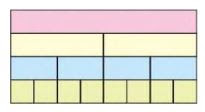How many one-fourths will make a half? How many 1/8 will make 1/4? How many 1/8 are in 1/2? Now ask your friends some questions on the same picture.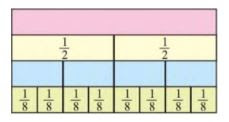The green strip is divided into 4 equal parts. Thus, each part is 1/4 of the whole green strip.

Two one-fourths will make a half.

1/4 + 1/4 = 2/4 =1/2 Two one-eighths will make 1/4 .

1/8 + 1/8 = 1 + 1/8 = 2/8 = 1/4

Disclaimer: The questions to be asked to friends may vary from student to student. It is highly recommended that the students prepare the answer on their own. The answer provided here is for reference only.

Question 2: Patterns Look at this square. What part is coloured blue? What part is green?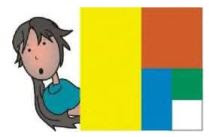We will draw a grid to find the portion of each colour in the following square.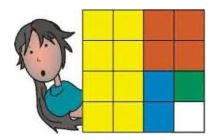Total number of small squares in the grid = 16

Number of small blue coloured squares = 2

Fraction of blue shaded square = 2/16 = 1/8

Thus, one-eighth of the square is shaded blue.

Number of green shaded squares = 1

Fraction of green shaded square= 1/16

Thus, one-sixteenth of the square is shaded green.

Question 3: Ammini says half of half and one-third of three-quarters are equal. Do you agree? How will you show this?

We can show the above with the help of figures.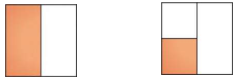Half                                         Half of half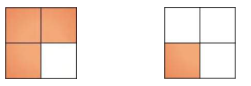3 quarters                                one third of 3 quarters

So, half of half is equal to one-quarter, and one-third of three-quarters is equal to one-quarter. Thus, we can say that half of half is equal to one- third of three quarters.

Page No 65:

Question 1: This show 1/5 petals of a flower. Complete the flower by drawing the other petals.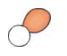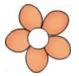Question 2: The picture shows one-third of the blades of a fan. Complete the picture by drawing the other blades.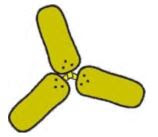Question 3: Half of the blades of another fan are shown here. Complete the picture by drawing the other half. How many blades have you drawn?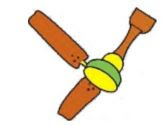We have drawn two more blades to complete the picture.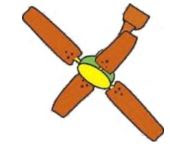Question 4:

will make one rupee? Is 50 paise half of one rupee?

will make one rupee?

25 paise is _______ part of one rupee 20 paise is _______ part of one rupee How many 10 paise will make one rupee? So 10 paise is _______ part of one rupee.

We know that,

One rupee = 100 paise Number of 50 paise coins in one rupee = 100 ÷ 50 = 2

So, two 50 paise coin will make one rupee.

There are two 50 paise coin in one rupee. So, 50 paise is half of one rupee. We know that, 100 paise = Re 1 Number of 25 paise in one rupee = 100 ÷25 = 4 So, four 25 paise coins will make I rupee.

We know that, four 25 paise coins will make 1 rupee. So, 25 paise is one- fourth part of one rupee.

Number of 20 paise coin in one rupee = 100 ÷20=5

There are five 20 paise coin in one rupee. So, 20 paise is one-fifth part of one rupee.

Number of 10 paise coin in one rupee = 100 ÷10 = 10 Ten 10 paise coin will make one rupee.

So 10 paise is one-tenth part of one rupee.

Page No 66:

Question 1: An Old Woman's Will Once there lived an old woman. She lived with her three daughters. She was quite rich and had 19 camels. One day she fell ill. The daughters called the doctor. The doctor tried his best but could not save the woman. After her death, the daughters read what she had written in her will.

 My eldest daughter will get12 of my camels My second daughter will get      14 of my camels My third daughter will get15 of my camels

The daughters were really puzzled. "How can I get 12 of the 19 camels?" asked the eldest daughter. "Half of 19 is nine and a half. But we can't cut the camel!" The second daughter said. "That is right. But what will we do now?" asked the third daughter". Just then they saw their aunt coming. The daughters told her their problem. "Show me the will. I have an idea. You take my camel. So you have 20 camels. Now can you divide them as your mother wanted?" the aunt said. "You want half of the camels, don't you? Take 10 camels" she said to the eldest daughter. "Take your share", the aunt told the second daughter. She took one-fourth of the camels and got _______ camels. "You can take one-fifth of the camels", the aunt told the third daughter . She got _______ camels. The daughters were very happy and counted their camels 10 + ___ +  ___ = 19. "The one remaining is mine", said the aunt and took her camel away!

• How did this happen? Discuss.

Total number of camels = 20 Number of camels given to first daughter = 20 ÷2 = 10 Thus, first daughter got 10 camels in her share.

Number of camels given to second daughter = 20 ÷ 4 = 5 Thus, second daughter got 5 camels in her share.

Number of camels given to third daughter = 20 ÷ 5= 4 Thus, third daughter got 4 camels in her share.

10 + 5 + 4 = 19 When the daughters counted their camels, they found that there were in total 19 camels to be divided among them in total.

The division of 19 camels among 3 daughters in the given fractions was possible only when the aunt included her camel.

Page No 67:

Question 1:

Arun's Time Table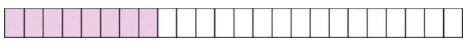Sleeping: One third of a day Use different colours to show Playing: One eight of a day Studying: 1/4 of a day

How many hours does Arun take for Sleeping?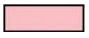hours Studying?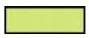hours Playing?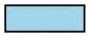hours What part of the day does he use for other activities?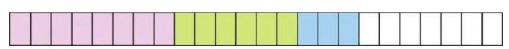Total number of hours in 1 day = 24 Arun sleeps one-third of a day. Number of hours that Arun sleeps = 24 ÷ 3=8

Thus, Arun sleeps for 8 hours in a day. Arun plays for one-eighth of a day. Number of hours Arun takes to play = 24 ÷ 8=3

Arun studies one-fourth of a day. Number of hours Arun takes to study 24 ÷ 4 = 6

Total number of hours spent in studying, playing and sleeping = 6+3+ 8 = 17 Number of hours left for other activities = 24 − 17=7

So, part of the day used for other activities = 7/24

Thus, Arun uses seven twenty-fourth of the day for other activities.

Page No 68:

Question 1: A school has decided to bring out a magazine every quarter of the year. How many magazines will they have in a year? If they want to print it at the end of each quarter of a year, which are the months for printing? Mark the number for those months.

 1 2 3 4 5 6 7 8 9 10 11 12

We know that,

1 year = 12 months Quarter of a year = 3 months Number of quarters in 1 year = 12 ÷ 3=4

A school brings 1 magazine every quarter of the year. Number of magazines brought by school in 1 year = 1 × 4 = 4

As the magazines are printed at the end of each quarter of the year, March, June, September and December will be the months for printing.

The given months are marked on the strip as: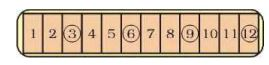Question 2:

Have you heard of Kumbhakarna, the brother of Ravana? He is famous for sleeping for half a year. Most people sleep about 8 hours a day. Then what part of a day is it? _______

So what part of a year do they sleep? A person 60 years old must have slept _______ years!!!

Total number of hours in one day = 24

Number of hours' people slept = 8

Part of the day people slept = 8/24 = 1/3

It is one-third part of a day.

Number of days in one ordinary year = 365

Number of hours in one day = 24

Number of hours in 365 days = 365 x 24 = 8760

Number of hours that people slept in 1 day = 8

Number of hours that people slept in 365 days = 365 × 8 = 2920

The part of the year that people slept  2920/ 8760 = 1/3  So, people slept one-third

of the year.

A man sleeps one-third part of a year. Number of years a man of 60 years slept = 60 ÷ 3=20

Thus, a man of 60 years must have slept 20 years.

Page No 69:

Question 1: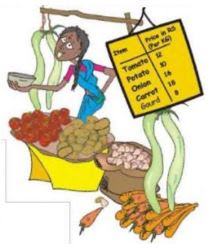Look at the yellow price list.

(a) How much does 2 kg of tomato cost?

(b) How much does 12 kg of tomato cost?

(c) Kiran wants 2 ½ kg of tomato. How much will it cost?

(d) How much does 3 ½  kg potato cost?

(e) What is the price of 1¼ kg of carrot?

(f) He bought a gourd of weight 4 ¾ kg and it costs _______

(g) Look at the shopping list in Keerti's hand. How much will she have to pay to buy all of these?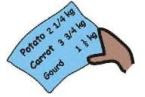(h) Make a bill of your own for vegetables you want to buy. Find the total money you will have to pay.

 Item Price in Rs. (per kg) Amount Total

a) Cost of 1 kg tomato = Rs 12 Cost of 2 kg tomatoes = Rs 2 x 12 = Rs 24

b) Cost of 1 kg tomato = Rs 12

Cost of 1/2 kg tomatoes = Rs 12 ÷ 2 = Rs 6

c) Cost of 1 kg tomato = Rs 12 So, cost of 2 ½ kg tomatoes = Rs 12+ Rs

12 +Rs 6 = Rs 30

d) Cost of 1 kg potato = Rs 10

Cost of 1/2 kg potato = Rs 10 ÷ 2 = Rs 5

Cost of 2 ½  kg potato = Rs 10 + Rs 10 + Rs 5 = Rs 25

e) Cost of 1 kg carrot = Rs 18

Cost of 1/4 kg carrot = Rs 18 ÷ 4= Rs = 18/4

Cost of  1¼ kg carrot = Rs 18+ Rs 18/4 = 9/2

f) Cost of 1 kg gourd = Rs 8

Cost of 3/4 kg gourd = Rs 6

Cost of 4 ¾ kg gourd = Rs 8 x 4 + Rs 6 = Rs 32 + Rs 6 = Rs 38

g) Cost of 1 kg potato = Rs 10

Cost of 1/4 kg potato = Rs 10 ÷ 4 = Rs  10/4

Cost of 2 ¼  kg potato = Rs 10 × 2 + Rs 10/4 = Rs 94 x 10 = Rs 452 = Rs 22/12

h)

 Item Price in Rs. (per kg) Amount Potato( 3 ½ kg ) 30 Rs. 105 Tomato( 3 kg) 40 Rs. 120 Cabbage ( 1 ¼ kg) 20 Rs. 25 Total Rs. 250

Page No 70:

Question 1: Raheem's journey- Raheem has to travel 1 ¼ km to reach school. What distance does he travel to go to school and come back home?

Distance travelled by Raheem to reach school from home = 1 ¼ km

Distance travelled by Raheem to return home from school = 1 ¼ km

Total distance travelled by Raheem =

1 ¼ + 1 ¼ = 1 + 1 + 1/4 + 1/4 = 2 + 2/4 = 2 + 1/2  = 2 ½ km Thus, the total distance travelled by Raheem to go and return from school is 2 ½ km.

Question 2: What coins? Latha bought a pencil and a pen for seven and a half rupees. She gave Rs 10/-. The shopkeeper gave back the money in half and quarter rupees. What are the coins she got?

Cost of a pen and a pencil = Rs 7 ½

Total money given to the shopkeeper = Rs 10

Total money returned to Latha by the shopkeeper = Rs 10 − Rs 7 ½ = 10 − ½ =3 − ½  = Rs 2 ½

We know that, 1 quarter rupee = 25 paise 1 half rupee = 50 paise

Now, the shopkeeper can return Rs 2 ½ in the following ways:

a) I half rupee coin and 8 quarter rupee coins

b) 4 half rupee coins and 2 quarter rupee coins

c) 2 half rupee coins and 6 quarter rupee coins

d) 3 half rupee coins and 4 quarter rupee coins

Question 3: At the railway station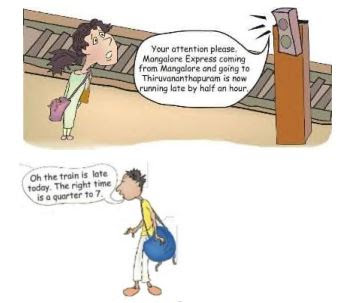(a) What time is the train expected to come today?

(b) Nazia gets off at a station after 2 ½ hours from this station. What time will she get off?

(c) Shaji will take 5 hours to reach Ernakulam by this train. At what time will he reach there?

a) The correct time for the arrival of the train is quarter to 7 = 6:34hrs But, the train is late by half an hour.

So, expected time of the arrival of the train

The right time is a quarter to 7 i.e. 6: 45

But, train is delayed by half an hour i.e. 30 minutes.

So, exact time train will arrive at 6: 45 + 0:30 =7: 14

Thus, the expected time of the arrival of the train is quarter past 7.

b) Expected time of the arrival of the train = 7: 14 hrs.

Nazia gets off at a station after 2 ½ hrs. of boarding the train.

Time at which Nazia will get off=

From the question,

Nazia gets off at a station after = 2:30 hours

Then, total time taken by Nazia to reach = 2: 30 +7: 15 = 9:45

Thus, Nazia will get off from the train at quarter to 10.

c) Shaji will reach Ernakulam by this train after 5 hours.

Expected time of the arrival of the train = 7: 14hrs

Expected time at which Shazi will reach Ernakulam =

From the question, it is given that,

Shaji will take 5 hours to reach Ernakulam

Then, total time taken by Shaji to reach Ernakulam = 7: 15 + 5 = 12: 15

Thus, Shazi will reach Ernakulam at quarter past 12.